Want to share your content on R-bloggers? click here if you have a blog, or here if you don't.

This blog post is about my recently released package on CRAN , OpenImageR. The package supports functions for image pre-processing, filtering and image recognition and it uses RccpArmadillo extensively to reduce the execution time of computationally intesive functions. OpenImageR can be split in 3 parts : basic functions (convolution, cropImage, down_sample_image, flipImage, gamma_correction, imageShow, image_thresholding, List_2_Array, MinMaxObject, NormalizeObject, readImage, resizeImage, rgb_2gray, rotateFixed, rotateImage, writeImage), image filtering (Augmentation, delationErosion, edge_detection, translation, uniform_filter, ZCAwhiten) and image recognition (average_hash, dhash, hash_apply, HOG, HOG_apply, invariant_hash, phash). The following code snippets explain the functionality of the OpenImageR package in more detail,

## Basic functions

The readImage function reads images from a variety of types such as ‘png’, ‘jpeg’, ‘jpg’ or ‘tiff’,

```library(OpenImageR)

path = 'image1.jpeg'           # path to an existing image

dim(im)

##  496 487   3
```

#### imageShow

The imageShow function utilizes either a shiny application (if the image is a character path) or the grid.raster function of the base grid package (if the image is a 2- or 3-dimensional object) to display images,

```imageShow(im)
```#### writeImage

The writeImage function writes a 2- or 3-dimensional object (matrix, data frame or array) in a user specified image format. The supported types are .png, .jpeg, .jpg, .tiff.

```writeImage(im, file_name = 'my_image.jpeg')
```

#### rgb_2gray

To convert an image from RGB to gray one can use the rgb_2gray function, which takes a single argument (matrix, data frame or array),

```path = 'image2.jpg'

r2g = rgb_2gray(im1)

imageShow(r2g)
```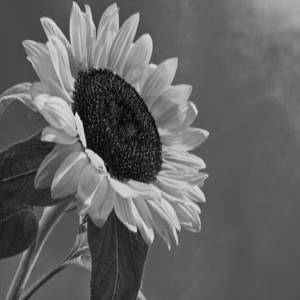#### cropImage

The cropImage function reduces the size of the image horizontally and vertically. The function takes four arguments : image (2- or 3-dimensional object), new_width (the desired new width), new_height (the desired new height) and type. While the type ‘equal_spaced’ crops the image equally in both directions (horizontal, vertical) towards the center of the image, the type ‘user_defined’ allows the user to specify which regions of the image should be kept

```us_def = cropImage(im1, new_width = 10:170, new_height = 15:190, type = 'user_defined')

imageShow(us_def)
```#### flipImage

A flipped image (or reversed image) is a static or moving image that is generated by a mirror-reversal of an original across a horizontal axis (a flopped image is mirrored across the vertical axis). The first image shows a horizontal flip,

```flp_horiz = flipImage(im, mode = 'horizontal')

imageShow(flp_horiz)
```whereas the second image a vertical flip,

```flp_vert = flipImage(im, mode = 'vertical')

imageShow(flp_vert)
```#### rotateImage

There are two functions available in the OpenImageR package to rotate an image : rotateFixed and rotateImage. The first one rotates an image by a fixed angle (90, 180, 270), whereas the second allows the user to rotate an image arbitrarily (between 0 and 360 radians) utilizing either the ‘nearest’ or the ‘bilinear’ interpolation method. An advantage of the first over the second function is that rotateFixed executes slightly faster.

```r45 = rotateImage(im, 45, threads = 1)

imageShow(r45)
```#### resizeImage

The resizeImage function takes advantage of two different interpolation methods ( nearest, bilinear ) to either down- or upsample an image,

```intBl = resizeImage(im, width = 100, height = 100, method = 'bilinear')

imageShow(intBl)
```It is known that resizing an image using bilinear interpolation gives better results, however the nearest neighbors method returns the output faster.

A further option to resize an image is by using gaussian_blur ( down-sampling gives better results than up-sampling that’s why is the only option in the OpenImageR package ),

```intGbl = down_sample_image(im, factor = 2.5, gaussian_blur = T)

imageShow(intGbl)
```The factor of the function applies equally to the horizontal and vertical dimensions of the image.

#### translation

translation is the shifting of an object’s location by adding/subtracting a value to/from the X or Y coordinates,

```tr = translation(im, shift_rows = 35, shift_cols = -40)

imageShow(tr)
```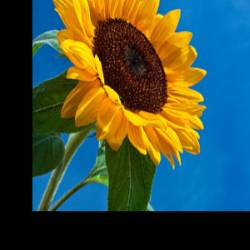here, shift_rows and shift_cols correspond to the rows and columns of the image.

## Image filtering

#### edge_detection

According to Wikipedia, edge detection is the name for a set of mathematical methods which aim at identifying points in a digital image at which the image brightness changes sharply or, more formally, has discontinuities. The edge_detection function uses one of the Frei_chen, LoG (Laplacian of Gaussian), Prewitt, Roberts_cross, Scharr or Sobel filters to perform edge detection,

```edsc = edge_detection(im, method = 'Scharr', conv_mode = 'same')

imageShow(edsc)

```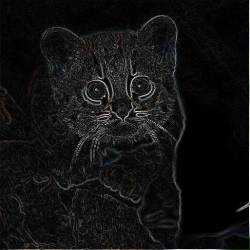#### uniform_filter

In a uniform filter all the values within the filter (kernel) have the same weight. An example of a uniform kernel is the unif_filt matrix,

```kernel_size =  c(4,4)

unf = uniform_filter(im, size = kernel_size, conv_mode = 'same')

unif_filt = matrix(1, ncol = kernel_size, nrow = kernel_size)/(kernel_size * kernel_size)
unif_filt

##        [,1]   [,2]   [,3]   [,4]
## [1,] 0.0625 0.0625 0.0625 0.0625
## [2,] 0.0625 0.0625 0.0625 0.0625
## [3,] 0.0625 0.0625 0.0625 0.0625
## [4,] 0.0625 0.0625 0.0625 0.0625

```

#### image_thresholding

According to Wikipedia, thresholding is the simplest method of image segmentation. Thresholding can be used to transfrom a grayscale image to a binary one,

```thr = image_thresholding(im, thresh = 0.5)

imageShow(thr)
```#### gamma_correction

Gamma correction, or often simply gamma, is the name of a nonlinear operation used to encode and decode luminance or tristimulus values in video or still image systems (Wikipedia),

```gcor = gamma_correction(im, gamma = 2)        # image with gamma correction

imageShow(gcor)
```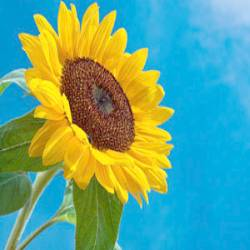#### ZCAwhiten

Whitening (or sphering) is the preprocessing needed for some algorithms. If we utilize an algorithm to train on images, the raw input is redundant, since adjacent pixel values are highly correlated. The purpose of whitening is to reduce the correlation between features and to return features with the same variance,

```res = ZCAwhiten(im, k = 20, epsilon = 0.1)
```

#### delationErosion

Dilation and erosion are the most basic morphological operations. Dilation adds pixels to the boundaries of objects in an image,

```res_delate = delationErosion(im, Filter = c(8,8), method = 'delation')

imageShow(res_delate)
```while erosion removes pixels on object boundaries,

```res_erosion = delationErosion(im, Filter = c(8,8), method = 'erosion')

imageShow(res_erosion)
```#### Augmentation

Augmentations are specific transformations applied to an image. The Augmentation function allows the user to flip, crop, resize, shift, rotate, zca-whiten and threshold an image. It either returns a single image,

```augm = Augmentation(im, flip_mode = 'horizontal', crop_width = 20:460, crop_height = 30:450,

resiz_width = 180, resiz_height = 180, resiz_method = 'bilinear',

shift_rows = 30, shift_cols = -40, rotate_angle = 350,

rotate_method = 'bilinear', zca_comps = 0,

zca_epsilon = 0.0, image_thresh = 0.0, threads = 1, verbose = T)

##
## time to complete : 0.03701949 secs

imageShow(augm)
```or multiple transformed images using either pre-specified or random parameters (here I utilize the lapply function),

```# random rotations
samp_rot = sample(c(seq(5, 90, 30), seq(270, 350, 30)), 3, replace = F)

# random shift of rows
samp_shif_rows = sample(seq(-50, 50, 10), 3, replace = F)

# random shift of columns
samp_shif_cols = sample(seq(-50, 50, 10), 3, replace = F)

res = lapply(1:length(samp_rot), function(x)

Augmentation(im, flip_mode = 'horizontal', crop_width = 20:460, crop_height = 30:450,

resiz_width = 180, resiz_height = 180, resiz_method = 'bilinear',

shift_rows = samp_shif_rows[x], shift_cols = samp_shif_cols[x],

rotate_angle = samp_rot[x], rotate_method = 'bilinear', zca_comps = 100,

zca_epsilon = 0.1, image_thresh = 0.0, threads = 1, verbose = F))
```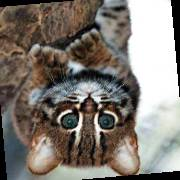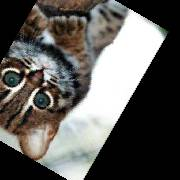## Image recognition

#### HOG ( histogram of oriented gradients )

The histogram of oriented gradients (HOG) is a feature descriptor used in computer vision and image processing for the purpose of object detection. The technique counts occurrences of gradient orientation in localized portions of an image. This method is similar to that of edge orientation histograms, scale-invariant feature transform descriptors, and shape contexts, but differs in that it is computed on a dense grid of uniformly spaced cells and uses overlapping local contrast normalization for improved accuracy (Wikipedia).

The HOG function of the OpenImageR package is a modification and extention of the findHOGFeatures function ( SimpleCV python library ), please consult the COPYRIGHTS file.

The purpose of the function is to create a vector of HOG descriptors, which can be used in classification tasks. It takes either RGB (they will be converted to gray) or gray images as input,

```image = readImage('image2.jpg')

image = image * 255

hog = HOG(image, cells = 3, orientations = 6)
hog

##   0.77467384 1.14086398 2.31882081 1.23274428 2.11970154 2.18883489
##   0.98549496 2.01821812 1.45391358 1.57411171 1.30782294 1.46338406
##  2.02594883 1.34298808 0.67503592 2.00982150 1.17643072 0.45404311
##  1.88299504 0.84538174 0.91915237 1.57228604 1.63834997 0.71412237
##  1.02929321 2.34486369 1.87591134 1.29050657 3.25947824 1.45813862
##  0.72216189 1.31668291 2.10979527 0.69908412 0.44783304 1.65000416
##  0.47910579 0.43018401 0.30561757 0.15398813 0.88550213 0.25647707
##  0.49130379 0.29065789 0.17552517 0.09544067 0.25962132 0.48733020
##  0.52154428 0.61910760 0.03944711 0.05271813 0.04068158 0.15660130
```

#### HOG_apply

The HOG_apply function uses the previous mentioned HOG function to return the HOG-descriptors for the following objects :

• a matrix of images such as the mnist data set, where each row represents a different digit (28 x 28 image)
• an array, where each slice represents a different image
• a folder of images where each file is a different image

In the following code chunk I’ll apply the HOG function to an array of images. The result is a matrix, where each row respresents the HOG-descriptors for each array slice (image),

```tmp_im1 = readImage('image1.jpeg')

tmp_im1 = resizeImage(tmp_im1, 200, 200)
tmp_im2 = resizeImage(tmp_im2, 200, 200)

tmp_gray1 = rgb_2gray(tmp_im1)
tmp_gray2 = rgb_2gray(tmp_im2)
dim(tmp_gray2)

##  200 200

tmp_arr = array(0, c(nrow(tmp_gray1), ncol(tmp_gray1), 2))
tmp_arr[,,1] = tmp_gray1
tmp_arr[,,2] = tmp_gray2

res = HOG_apply(tmp_arr, cells = 2, orientations = 3)

##
## time to complete : 0.01445627 secs

res

##            [,1]       [,2]       [,3]       [,4]       [,5]       [,6]
## [1,] 0.03480197 0.04194668 0.04618026 0.04136810 0.04315453 0.04107919
## [2,] 0.02451927 0.03900991 0.03382324 0.02727066 0.03311867 0.02102830
##            [,7]       [,8]       [,9]      [,10]      [,11]      [,12]
## [1,] 0.03416017 0.03931556 0.04142622 0.03464385 0.02948044 0.02239715
## [2,] 0.01256172 0.01003701 0.01500640 0.01639548 0.01053654 0.01457501

```

### image hashing

The image hashing functions (average_hash, dhash, phash, invariant_hash, hash_apply) of the OpenImageR package are implemented in the way perceptual hashing works. Perceptual hashing is the use of an algorithm that produces a fingerprint of images (in OpenImageR those fingerprints are binary features or hexadecimal hashes). The difference between cryptographic and image hashing is that the latter tries to find similar and not exact matches. In cryptographic hashing small differences of the hashes lead to entirely different output, which is not the case for perceptual hashing. A practical application of image hashing would be to compare a database of already created image hashes with a new hash (image) to find similar images in the database.

The average_hash, dhash, phash functions of the OpenImageR package are modifications and extentions of the ImageHash python library, please, consult the COPYRIGHTS file.

#### average_hash

The average hash algorithm of the OpenImageR package works in the following way : 1st we convert to grayscale, 2nd we reduce the size of an image (for instance to an 8×8 image), 3rd we average the resulting colors (for an 8×8 image we average 64 colors), 4th we compute the bits by comparing if each color value is above or below the mean and 5th we construct the hash. The result of the average_hash function can be either a hexadecimal hash (string) or binary features,

```image = readImage('view1.jpg')

imageShow(image)

image = rgb_2gray(image)

aveg_hash = average_hash(image, hash_size = 8, MODE = 'hash', resize = "bilinear")
aveg_hash

##  "ffffffde08000000"

aveg_bin = average_hash(image, hash_size = 8, MODE = 'binary', resize = "bilinear")
as.vector(aveg_bin)

##   1 1 1 1 1 1 1 1 1 1 1 1 1 1 1 1 1 1 1 1 1 1 1 1 0 1 1 1 1 0 1 1 0 0 0
##  1 0 0 0 0 0 0 0 0 0 0 0 0 0 0 0 0 0 0 0 0 0 0 0 0 0 0 0 0
```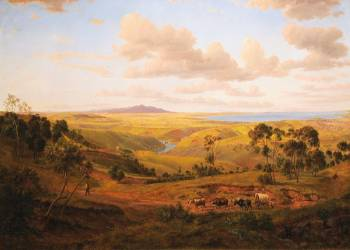#### phash

While the average_hash is fast, it can generate false-misses if there is a gamma correction or a color histogram is applied to the image. The phash algorithm extends the average_hash by using the discrete cosine transform to reduce the frequencies,

```image2 = readImage('view2.jpg')

imageShow(image2)

image2 = rgb_2gray(image2)

ph_hash = phash(image2, hash_size = 8, highfreq_factor = 4, MODE = 'hash', resize = "bilinear")
ph_hash

##  "5b63ecacb1a258c9"

ph_bin = phash(image2, hash_size = 8, highfreq_factor = 4, MODE = 'binary', resize = "bilinear")
as.vector(ph_bin)

##   1 1 0 1 1 0 1 0 1 1 0 0 0 1 1 0 0 0 1 1 0 1 1 1 0 0 1 1 0 1 0 1 1 0 0
##  0 1 1 0 1 0 1 0 0 0 1 0 1 0 0 0 1 1 0 1 0 1 0 0 1 0 0 1 1

```#### dhash

In comparison to average_hash and phash, the dhash algorithm takes into consideration the difference between adjacent pixels. In the same way as with the average_hash, the resulting hash won’t change if the image is scaled or the aspect ratio changes. Increasing or decreasing the brightness or contrast, or even altering the colors won’t dramatically change the hash value. Even complex adjustments like gamma corrections and color profiles won’t impact the result,

```image3 = readImage('view3.jpg')

imageShow(image3)

image3a = rgb_2gray(image3)

dh_hash = dhash(image3a, hash_size = 8, MODE = 'hash', resize = "bilinear")
dh_hash

##  "efbf63003c008012"

dh_bin = dhash(image3a, hash_size = 8, MODE = 'binary', resize = "bilinear")
as.vector(dh_bin)

##   1 1 1 1 0 1 1 1 1 1 1 1 1 1 0 1 1 1 0 0 0 1 1 0 0 0 0 0 0 0 0 0 0 0 1
##  1 1 1 0 0 0 0 0 0 0 0 0 0 0 0 0 0 0 0 0 1 0 1 0 0 1 0 0 0
```By adding gamma correction we can check if the hash value or the binary features of the view3.jpg will be altered,

```tmp_image3 = gamma_correction(image3, gamma = 0.5)

imageShow(tmp_image3)

tmp_image3 = rgb_2gray(tmp_image3)

dh_hash_a = dhash(tmp_image3, hash_size = 8, MODE = 'hash', resize = "bilinear")
dh_hash_a

##  "efbf63003c008012"

dh_bin_a = dhash(tmp_image3, hash_size = 8, MODE = 'binary', resize = "bilinear")
as.vector(dh_bin_a)

##   1 1 1 1 0 1 1 1 1 1 1 1 1 1 0 1 1 1 0 0 0 1 1 0 0 0 0 0 0 0 0 0 0 0 1
##  1 1 1 0 0 0 0 0 0 0 0 0 0 0 0 0 0 0 0 0 1 0 1 0 0 1 0 0 0
```#### invariant_hash

invariant_hash is an extension function for image hashing. It takes two images as input (image1, image2) and by altering one of those (random flipping, rotating and cropping) it calculates the hamming distance (if the mode is ‘binary’) or the levenshtein distance if (the mode is ‘hash’). If any of the flip, rotate, crop equals TRUE then the function returns the MIN,MAX similarity between the two images. If, on the other hand all flip, rotate, crop equal FALSE then a single similarity value is returned meaning no random transformations of the second image are performed.

Although, in the previous example the gamma correction doesn’t influence the dhash of the view3.jpg, a horizontal flip of the image would change both the hash value and the binary features considerably,

```image3b = flipImage(image3, mode = "horizontal")

imageShow(image3b)

image3b = rgb_2gray(image3b)

dh_hash_b = dhash(image3b, hash_size = 8, MODE = 'hash', resize = "bilinear")
dh_hash_b

##  "fffe63001e8002a4"

dh_bin_b = dhash(image3b, hash_size = 8, MODE = 'binary', resize = "bilinear")
as.vector(dh_bin_b)

##   1 1 1 1 1 1 1 1 0 1 1 1 1 1 1 1 1 1 0 0 0 1 1 0 0 0 0 0 0 0 0 0 0 1 1
##  1 1 0 0 0 0 0 0 0 0 0 0 1 0 1 0 0 0 0 0 0 0 0 1 0 0 1 0 1
```By using the invariant hash in this case with all the available transformations (at the cost of computational time) we can obtain minimum and maximum values for the hamming or the levenshtein distance,

```inv_hash = invariant_hash(image3a, image3b, mode = 'binary', flip = T, rotate = T,

angle_bidirectional = 10, crop = T)
inv_hash

##   min     max
## 1   0 0.53125

inv_bin = invariant_hash(image3a, image3b, mode = 'hash', flip = T, rotate = T,

angle_bidirectional = 10, crop = T)
inv_bin

##   min max
## 1   0  15
```

In both cases (hash, binary) a minimum value of 0 indicates perfect matches between the two images among the transfomations.

#### hash_apply

The hash_apply function applies the single average_hash, phash, dhash to either a matrix, array or folder of images. This function is practical in case of a matrix of images such as the mnist, or the cifar_10 data sets, where each line of the matrix corresponds to an image. Furthermore, if a folder includes many images, then the hexadecimal hash mode should be prefered, as it doesn’t require as much storage space as the binary mode. The following example illustrates how hash values and binary features can be computed from a folder of images,

```path = paste0(getwd(), '/TEST_hash/')

hapl_hash = hash_apply(path, hash_size = 6, method = "dhash", mode = "hash", threads = 1,  resize = "nearest")

##
## time to complete : 0.009102345 secs

hapl_hash                # returns both the names of the images and the hash values

## \$files
##   "2_1.png" "2_2.png" "2_3.png" "4_1.png" "4_2.png" "4_3.png" "5_1.png"
##   "5_2.png" "5_3.png" "8_1.png" "8_2.png" "8_3.png" "9_1.png" "9_2.png"
##  "9_3.png"
##
## \$hash
##   "00808310" "00808310" "00808310" "0080a024" "0080a024" "0080a024"
##   "00002308" "00002308" "00002308" "00801108" "00801108" "00801108"
##  "3e00200c" "3e00200c" "3e00200c"

hapl_bin = hash_apply(path, hash_size = 6, method = "dhash", mode = "binary", threads = 1,  resize = "nearest")

##
## time to complete : 0.008812904 secs

hapl_bin\$files             # names of the images

##   "2_1.png" "2_2.png" "2_3.png" "4_1.png" "4_2.png" "4_3.png" "5_1.png"
##   "5_2.png" "5_3.png" "8_1.png" "8_2.png" "8_3.png" "9_1.png" "9_2.png"
##  "9_3.png"

dim(hapl_bin\$hash)         # dimensions of the resulted matrix

##  15 36

##      [,1] [,2] [,3] [,4] [,5] [,6] [,7] [,8] [,9] [,10] [,11] [,12] [,13]
## [1,]    0    0    0    0    0    0    0    0    0     0     0     0     0
## [2,]    0    0    0    0    0    0    0    0    0     0     0     0     0
## [3,]    0    0    0    0    0    0    0    0    0     0     0     0     0
## [4,]    0    0    0    0    0    0    0    0    0     0     0     0     0
## [5,]    0    0    0    0    0    0    0    0    0     0     0     0     0
## [6,]    0    0    0    0    0    0    0    0    0     0     0     0     0
##      [,14] [,15] [,16] [,17] [,18] [,19] [,20] [,21] [,22] [,23] [,24]
## [1,]     0     0     1     1     1     0     0     0     0     0     1
## [2,]     0     0     1     1     1     0     0     0     0     0     1
## [3,]     0     0     1     1     1     0     0     0     0     0     1
## [4,]     0     0     1     0     0     0     0     0     1     0     1
## [5,]     0     0     1     0     0     0     0     0     1     0     1
## [6,]     0     0     1     0     0     0     0     0     1     0     1
##      [,25] [,26] [,27] [,28] [,29] [,30] [,31] [,32] [,33] [,34] [,35]
## [1,]     0     0     0     0     1     0     0     0     1     1     1
## [2,]     0     0     0     0     1     0     0     0     1     1     1
## [3,]     0     0     0     0     1     0     0     0     1     1     1
## [4,]     0     0     1     0     0     1     0     0     1     1     1
## [5,]     0     0     1     0     0     1     0     0     1     1     1
## [6,]     0     0     1     0     0     1     0     0     1     1     1
##      [,36]
## [1,]     0
## [2,]     0
## [3,]     0
## [4,]     0
## [5,]     0
## [6,]     0
```

An updated version of the OpenImageR package can be found in the Github repository and to report bugs/issues please use the following link, https://github.com/mlampros/OpenImageR/issues.